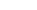•WE PROVIDEMATH
HOMEWORK
HELP
We can help with
Algebra
Calculus
Statistics
and any Math subject
starts at \$35/hour.

# Get Math Homework Help

• Algebra
• Linear Equations
• Solving Inequalities
• Absolute Value

• Calculus
• Functions
• Limits
• Continuity
• Derivatives
• Chain Rule, Related Rates
• Integration

• Statistics
• Descriptive Statistics
• Confidence Intervals
• Hypothesis Testing
• ANOVA
• Linear Regression

• Economics
• Demand and Supply
• Perfect Competition
• Elasticity
• Monopolistic Competition
• Oligopolies
• Monopolies

• Linear Algebra
• Matrices
• Determinants
• Vector Spaces
• Linear Independence

• Operations Management
• Time Series
• Forecasts
• Inventory Models
• Decision Theory
• Linear Programming
• Waiting Lines

## Claim Your Free Solved Stats Problems!

* indicates required
Email Format

## Our Specialties

#### Inequalities

Solving complex inequalities can be challenging. Our Math experts are extremely proficient and familiar with all types of inequality problems presented in the most common Math and Algebra Textbooks, so they can definitely help you with that.

#### Word Problems

We get help requests all the time for complex word problems. Don't get lost and get an expert to help you.

#### Calculus

This is a tough one for most students, Your Calculus class could of the the most challenging points in your academic career. Calculus homework problems usually involve working with functions, getting intercepts, asymptotes, maxima and minima, inflection points and a very accurate of all these elements together. We can help you that too.

#### System of Equations

Dealing with systems of equations can be tricky, as there are different methods that can be used and you sometimes are asked for one specific method. We can help YOU with all types of systems of equations.